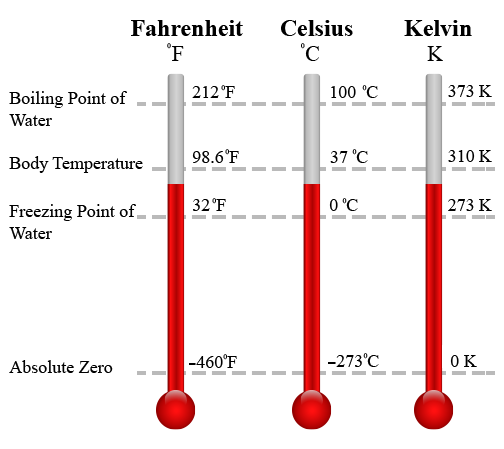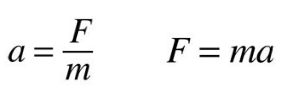# ASVAB Science Study Guide Part 3

## Physical Science

This is the third part of our free ASVAB Science Study Guide. This section of the study guide covers temperature, physics, and energy. These are important topics that are very likely to show up on your ASVAB test.

### Temperature

There are three temperature scales that are commonly used today: Fahrenheit, Celsius and Kelvin.

Fahrenheit is the official temperature scale of the United States, and is used by a few other countries as well. The Fahrenheit scale is based on 32° as the freezing point of water and 212° as the boiling point of water.

Celsius is the temperature scale used by almost every country besides the US. It is the scale which is incorporated into the International System of Units (SI). The Celsius scale is based on 0° as the freezing point of water and 100° as the boiling point of water. It is sometimes called the centigrade scale because of the 100-degree interval between the two defined points.

Kelvin is a temperature scale that is sometimes used in science and engineering. The lowest temperature in Kelvin is 0 K, which indicates absolute zero. Absolute zero is the point at which all thermal motion ceases. The boiling point of water is set at 373.15 K. Unlike Fahrenheit and Celsius, Kelvin is not referred to or written in degrees.## Physics

### Newton’s Laws

Newton’s first law of motion: An object at rest stays at rest and an object in motion stays in motion with the same speed and in the same direction unless acted upon by an unbalanced force. For example, a ball resting on the grass will remain resting on the grass until a force is applied, such as a foot. After the kicked ball begins rolling, it will continue to roll until a force (friction) causes it to slow down. Newton’s first law of motion is also called the law of inertia. Inertia is the tendency of an object to resist change in motion.

Newton’s second law of motion: The acceleration of an object is equal to the net force acting on it divided by the object’s mass. According to this law, acceleration is produced when a force acts on a mass. The greater the mass of the object being accelerated, the greater amount of the force needed to make the object accelerate. This is why it takes more force to move heavier objects the same distance as lighter objects. Using this law, acceleration can be calculated as follows:Newton’s third law of motion: For every action, there is an equal and opposite reaction. In other words, when one object exerts a force on a second object, the second object exerts an equal and opposite force on the first object. The two forces are called action and reaction forces. The force of your bumper car on the other bumper car when you crash into it is the action force. The force of the other bumper car on your car is the reaction force.

Newton’s law of universal gravitation: Isaac Newton did not discover gravity, but he was the first scientist to precisely define gravitational force, and to show that it could explain falling objects as well as astronomical motions. He discovered that all objects attract each other through a universal force of gravitational attraction. The strength of this gravitational force depends on the masses of the objects and the distance that separates their centers. More precisely, his formula states that the gravitational force between two objects is directly proportional to the product of their masses and inversely proportional to the square of the distance between them.

### The Speed of Light & Sound

Speed of Light: 300,000 kilometers per second, or 186,000 miles per second.

Speed of Sound: 343 meters per second (in air), or 4.7 seconds to travel 1 mile.

The speed of sound is 4.3 times faster when travelling through water.

Albert Einstein developed the Theory of Special Relativity which shows that the laws of physics are the same for all non-accelerating observers, and that the speed of light within a vacuum is the same no matter the speed at which an observer travels. This showed that space and time are interwoven into a single continuum known as space-time. Events occurring at the same time for one person could occur at different times for another observer.

### Kinetic Energy vs. Potential Energy

Energy can be classified into two general types: kinetic energy and potential energy.

Kinetic energy: The energy that an object possesses due to its motion.  Some examples of kinetic energy include a thrown baseball, a flying airplane, and a flowing river.

Potential energy: The energy stored by an object as a result of its position. Some examples of potential energy include a compressed spring, a lifted weight, and a stretched rubber band.

### Forms of Energy

All energy forms are either potential or kinetic, but there are classifications that can be made within these categories. Potential energy comes in forms that are stored including nuclear, chemical, mechanical, and gravitational. Kinetic energy comes from different types of movement including electrical energy, light, sound, and heat.

Forms of Potential Energy
Mechanical energy is energy stored in objects by tension.

Examples: Stretched rubber bands, compressed springs.
Chemical energy is the energy stored within chemical bonds of atoms and molecules.

Examples: Batteries, petroleum, natural gas, coal.
Nuclear energy is the potential energy of the particles inside an atomic nucleus.

Examples: Nuclear fission and nuclear fusion.
Gravitational energy is the potential energy that is stored in an object’s height.

Examples: Hydro power, a bowling ball that is being held by someone.
Forms of Kinetic Energy
Thermal energy (heat) is the total potential and kinetic energy related to the motion of subatomic particles in an object.

Examples: A boiling pot of water, geothermal energy (heat from the earth).
Electrical energy is the energy resulting from the flow of an electric charge.

Examples: Lightning, electric current, electric eels.

Examples: Sunshine, x-rays.
Sound Energy is a form of energy produced from the vibration of an object. It can travel by transferring energy from one particle to other.

Examples: Car horn, drums, thunder.
Motion/Mechanical Energy is energy stored in the movement of objects. It’s the kinetic type of mechanical energy.

Examples: Wind, a moving car, a ball that’s been thrown.

## Part 3 Review Quiz:

Congratulations - you have completed .

You scored %%SCORE%% out of %%TOTAL%%.

Your performance has been rated as %%RATING%%

 Question 1

### At the temperature of 0 K, absolute zero,

 A Water reaches its boiling point B All thermal motion stops C Water reaches its freezing point D Solid objects break down
Question 1 Explanation:
Absolute zero is the lowest temperature that can be recorded in Kelvin. At this point, all thermal motion ceases.
 Question 2

### Decreasing the force behind an object will _______ the acceleration it experiences.

 A decrease B increase C remain the same D not enough information to tell
Question 2 Explanation:
Acceleration is calculated by dividing the force of an object by its mass. If the force is decreased but the mass remains unchanged, the acceleration will decrease as well.
 Question 3

### The strength of gravitational force depends on

 A the masses and the weight of each object B the masses of both objects C the mass of an object D the masses of each object and the distance between their centers
Question 3 Explanation:
The strength of gravitational force depends on the masses of the objects and the distance that separates their centers. Weight is just a measurement of gravitational force.
 Question 4

### The speed of _____ is faster than the speed of ____.

 A sound; light B light; sound C kinetic energy; potential energy D thermal energy; gravitational pull
Question 4 Explanation:
Light travels at a speed of 186,000 miles per second, while sound takes 4.7 seconds to travel 1 mile, so it is even slower than one mile per second. Thus, light travels much, much faster than sound.
 Question 5

### A book that is sitting atop a high shelf is an example of

 A gravitational force B kinetic energy C potential energy D mechanical energy
Question 5 Explanation:
An object can have potential energy due to its height, as the book can fall from its shelf. This specific potential energy would be gravitational energy. This is very different from gravitational force. Mechanical energy is another form of potential energy, but it is due to built up tension, which a resting book does not have.
 Question 6

### ____ energy can be potential OR kinetic.

 A Thermal B Radiant C Mechanical D Sound
Question 6 Explanation:
Mechanical energy can come from built-up tension or an object's movement.
Once you are finished, click the button below. Any items you have not completed will be marked incorrect.
There are 6 questions to complete.
 ← List →